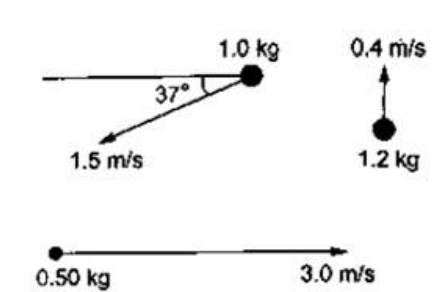# Solve the following :

Question:

Calculate the velocity of the center of mass of the system of particles shown in figure.Solution:

Velocity coordinates of $1 \mathrm{Kg} \rightarrow\left(-1.5 \cos 37^{\circ},-1.5 \sin 37^{\circ}\right)$

For another $1 \mathrm{Kg} \rightarrow\left(2 \cos 37^{\circ},-2 \sin 37^{\circ}\right)$

For $1.2 \mathrm{Kg} \rightarrow(0,0.4 \mathrm{~m} / \mathrm{s})$

For $1.5 \mathrm{Kg} \rightarrow\left(-1 \cos 37^{\circ}, 1 \sin 37^{\circ}\right)$

For $0.5 \mathrm{Ka} \rightarrow(3,0)$

$V_{x(\text { c.o.M })}=\frac{1\left(-1.5 \cos 37^{\circ}\right)+2 \cos 37^{\circ}+0-1.5 \cos 37^{\circ}+3 \times 0.5}{5.2}$

$=\frac{-\cos 37^{\circ}+1.5}{5.2}=0.13487 \mathrm{~m} / \mathrm{s}$

$V_{y(c . O . M)}=\frac{-1.5 \sin 37^{\circ}-2 \sin 37^{\circ}+(1.2 \times 0.4)+\sin 37^{\circ} \times 1.5+0}{5.2}$

$=\frac{-2 \sin 37^{\circ}+0.48}{5.2}=-0.13915 \mathrm{~m} / \mathrm{s}$

$\mathrm{COM}=(0.13487,-0.13915)$